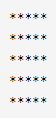Related Tags

java
communitycreator

# How to make a square star pattern in Java

Ayush Trivedi

In this shot, we will learn how to create a square star pattern using JavaScript. This pattern is the simplest among the other patterns. The logic to create a square pattern is easy to understand and implement.

A square star pattern looks like the image below:.This is a 5 x 5 square star pattern. You can see that we need to print * at every row and column. The logic for this is simple, you need a nested for loop which will loop for 5(n) times, along with the outer loop. At every i,j combination you have to print *.

### Code

let n = 5; // row or column count

for(let i = 0; i < n; i++) { // external loop
for(let j = 0; j < n; j++) { // internal loop
process.stdout.write('*');
}
// newline after each row
console.log();
}

### Explanation

• In line 1, we initialize the number of rows (the side) in the square.

• In line 3, the first for loop will iterate rows. We have five rows (n = 5) so it will loop five times.

• In line 4, this nested for loop will print * n times which is 5, because n = 5, this loop is responsible for printing the columns.

• In line 5, we print the * using standard method process.stdout.write('*').

• In line 8, after every row is completed we need to change the line. To get to the next line we use console.log() with a null value, which will print nothing and change to the next line.

RELATED TAGS

java
communitycreator

CONTRIBUTOR

Ayush Trivedi
RELATED COURSES

View all Courses

Keep Exploring

Learn in-demand tech skills in half the time